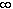#Interactive Real Analysis

Next | Previous | Glossary | Map

## 7.3. Measures

### Examples 7.3.15(a): Monotone sequences of measurable sets

For decreasing sets we had to assume that m(A1) was finite. Show that without this assumption the statement in the previous proposition is false.
We have to find a decreasing sequence of sets An such that lim m(An) is not equal to m(An).

Let An = [n,). Then m(An) =for all n, so in particular lim m(An) =.

On the other hand we have thatAn = 0 so that m(An ) = 0. That finishes the example.

Next | Previous | Glossary | Map